# Slides of the fifth lecture (Fractals and multifractals, 4739KB)

## Transcription

Slides of the fifth lecture (Fractals and multifractals, 4739KB)
```Dynamics and time series:
theory and applications
Stefano Marmi
Scuola Normale Superiore
Lecture 5, Feb 12, 2009
• Lecture 1: An introduction to dynamical systems and to time series. Periodic and
quasiperiodic motions. (Tue Jan 13, 2 pm - 4 pm Aula Bianchi)
• Lecture 2: Ergodicity. Uniform distribution of orbits. Return times. Kac inequality Mixing
(Thu Jan 15, 2 pm - 4 pm Aula Dini)
• Lecture 3: Kolmogorov-Sinai entropy. Randomness and deterministic chaos. (Tue Jan 27,
2 pm - 4 pm Aula Bianchi)
• Lecture 4: Time series analysis and embedology. (Thu Jan 29, 2 pm - 4 pm Dini)
• Lecture 5: Fractals and multifractals. (Thu Feb 12, 2 pm 4 pm Dini)
• Lecture 6: The rhythms of life. (Tue Feb 17, 2 pm - 4 pm Bianchi)
• Lecture 7: Financial time series. (Thu Feb 19, 2 pm - 4 pm Dini)
• Lecture 8: The efficient markets hypothesis. (Tue Mar 3, 2 pm - 4 pm Bianchi)
• Lecture 9: A random walk down Wall Street. (Thu Mar 19, 2 pm - 4 pm Dini)
• Lecture 10: A non-random walk down Wall Street. (Tue Mar 24, 2 pm – 4 pm Bianchi)
• Seminar I: Waiting times, recurrence times ergodicity and quasiperiodic
dynamics (D.H. Kim, Suwon, Korea; Thu Jan 22, 2 pm - 4 pm Aula Dini)
• Seminar II: Symbolization of dynamics. Recurrence rates and entropy (S.
Galatolo, Università di Pisa; Tue Feb 10, 2 pm - 4 pm Aula Bianchi)
• Seminar III: Heart Rate Variability: a statistical physics point of view (A.
Facchini, Università di Siena; Tue Feb 24, 2 pm - 4 pm Aula Bianchi )
• Seminar IV: Study of a population model: the Yoccoz-Birkeland model (D.
Papini, Università di Siena; Thu Feb 26, 2 pm - 4 pm Aula Dini)
• Seminar V: Scaling laws in economics (G. Bottazzi, Scuola Superiore
Sant'Anna Pisa; Tue Mar 17, 2 pm - 4 pm Aula Bianchi)
• Seminar VI: Complexity, sequence distance and heart rate variability (M.
Degli Esposti, Università di Bologna; Thu Mar 26, 2 pm - 4 pm Aula Dini )
• Seminar VII: Forecasting (M. Lippi, Università di
Roma; late april, TBA)
Self-similarity and fractals
A subset A of Euclidean space will be considered a “fractal”
when it has most of the following features:
• A has fine structure (wiggly detail at arbitrarily small
•
•
•
•
•
scales)
A is too irregular to be described by calculus (e.g. no
tangent space)
A is self-similar or self-affine (maybe approximately or
statistically)
the fractal dimension of A is non-integer
A may have a simple (recursive) definition
A has a “natural” appearance: “Clouds are not spheres,
mountains are not cones, coastlines are not circles, and
bark is not smooth, nor does lightning travel in a straight
line . . .” (B. Mandelbrot)
self-similar
fractals
self-affine
fractals
From: K. Falconer, Techniques in Fractal Geometry, Wiley 1997
self-conformal
fractals
Statistically
self-similar
fractals
From: K. Falconer, Techniques in Fractal Geometry, Wiley 1997
Source: Wikipedia
Source: Wikipedia
Mathematics, shapes and nature
http://classes.yale.edu/fractals/Panorama/Nature/NatFracGallery/Gallery/Gallery.html
From http://en.wikipedia.org/wiki/Image:Square1.jpg
Lichtenberg Figure
High voltage dielectric breakdown within a block of plexiglas creates a beautiful
fractal pattern called a Lichtenberg_figure. The branching discharges ultimately
become hairlike, but are thought to extend down to the molecular level.
Bert Hickman, http://www.teslamania.com
A diffusion-limited aggregation (DLA) cluster. Copper aggregate formed from
a copper sulfate solution in an electrode position cell. Kevin R. Johnson, Wikipedia
Coastlines
Massachusetts
D=1.15
Greece
D=1.20
200 km
100 km
http://upload.wikimedia.org/wikipedia/com
mons/2/20/Britain-fractal-coastlinecombined.jpg
50 km
How long is a coastline?
The answer depends on the scale at which the measurement is
made: if s is the reference length the coastline length L(s) will be
Log L(s) = (1-D) log s + cost
(Richardson 1961, Mandelbrot Science 1967)
How long is the coast of Britain?
Statistical self-similarity and fractional dimension
Science: 156, 1967, 636-638
B. B. Mandelbrot
Seacoast shapes are examples of highly involved curves with the
property that - in a statistical sense - each portion can be considered
a reduced-scale image of the whole. This property will be referred to
as „„statistical self-similarity.‟‟ The concept of „„length‟‟ is usually
meaningless for geographical curves. They can be considered
superpositions of features of widely scattered characteristic sizes; as
even finer features are taken into account, the total measured length
increases, and there is usually no clear-cut gap or crossover,
between the realm of geography and details with which geography
need not be concerned.
How long is the coast of Britain?
Statistical self-similarity and fractional dimension
Science: 156, 1967, 636-638
B. B. Mandelbrot
Quantities other than length are therefore needed to discriminate
between various degrees of complication for a geographical
curve. When a curve is self-similar, it is characterized by an
exponent of similarity, D, which possesses many properties of
a dimension, though it is usually a fraction greater that the
dimension 1 commonly attributed to curves. I propose to
reexamine in this light, some empirical observations in
Richardson 1961 and interpret them as implying, for example,
that the dimension of the west coast of Great Britain is D =
1.25. Thus, the so far esoteric concept of a „„random figure of
fractional dimension‟‟ is shown to have simple and concrete
applications of great usefulness.
“Box counting” dimension
s
N(s)
25
47
20
67
15
100
10
159
5
386
Log N(s) = -D log s + cost
http://www.physionet.org/tutorials/epn/program/coastline.htm
Box counting (Minkowski) dimension
Let E be a non-empty bounded subset of Rn and let Nr (E) be the
smallest number of sets of diameter r needed to cover E
• Lower dimension dimB E = liminfr→0 log Nr (E) / -logr
• Upper dimension dimB E = limsupr→0 log Nr (E) / -logr
• Box-counting dimension: if the lower and upper dimension
agree then we define
dim E = limr→0 log Nr (E) / -logr
The value of these limits remains unaltered if Nr (E) is taken to be
the smallest number of balls of radius r (cubes of side r)
needed to cover E, or the number of r-mesh cubes that
intersect E
Hausdorff dimension
A finite or countable collection of subsets {Ui } of Rn is a δcover of a set E if | Ui |< δ for all i and E is contained in Ui Ui
Hsδ (E) = inf {Σi | Ui |s , {Ui } is a a δ-cover of E}
s-dimensional Hausdorff measure of E: Hs (E) =limδ→0 Hsδ (E)
It is a Borel regular measure on Rn , it behaves well under
similarities and Lipschitz maps
The Hausdorff dimension dimH E is
the number at which the Hausdorff
measure Hs (E) jumps from ∞ to 0
dimH E ≤ dimB E ≤ dimB E
Von Koch curve (1904)
D=log4/log3=1.261859...
From: K. Falconer, Techniques in Fractal Geometry, Wiley 1997
Fractal snowflake
Infinite perimeter, finite area, D=log4/log3=1.261859...
Sierpinski triangle (1916)
D=log3/log2=1.5849625...
Source:
Wikipedia
A fractal carpet (zero area)
D=3log2/log3=1.892789...
A fractal sponge
Zooming in
Changing parameters
•The triangle of Sierpinski is the attractor of an iterated
function system (i.f.s).
•The i.f.s. is made of three affine maps (each contracting by a
factor ½ and leaving one of the initial vertices fixed)
•Combining the affine maps with rotations one can change the
shape considerably
90 anticlockwise rotation
about the top vertex
180 rotation about the
same vertex
Hausdorff metric and compact sets
E
F
Contractions and Hausdorff metric
Proposition: if w:X→X is a contraction with Lipschitz constant s
then w is also a contraction on (H (X),h) with Lipschitz
constant s
To each family F of contractions on X one can associate a
family of contractions on (H (X),h). By Banach-Caccioppoli
to each such F will correspond a compact nonempty subset A
of X: the attractor associated to F
d(w(E),w(F))=max min d(y,z) = max min d(w(e),w(f))
yϵE
zϵF
eϵE
fϵF
≤ s max min d(e,f) = s d(E,F)
eϵE
fϵF
Iterated function systems
F = {w1, …, wN} each wi : X→ X is a contraction of constant si,
0 ≤ si <1
Let W : H (X) → X
W (E) = U wi(E)
1≤i≤N
Then W contracts the Hausdorff metric h with Lipschitz constant
s = max si . We denote by A the corresponding attractor
1≤i≤N
Given any subset E of X, the iterates W ⁿ(E) → A exponentially
fast, in fact h(W ⁿ(E) , A ) ≈ sⁿ as n → ∞
Self similarity and fractal
dimension
If the contractions of the i.f.s. F = {w1, …, wN} are
• Similarities
the attractor A will be said self-similar
• Affine maps
the attractor A will be said self-affine
• Conformal maps (i.e. their derivative is a similarity) then the
attractor A will be said self-conformal
If the open set condition is verified, i.e. there exists an open set U
such that wi(U)∩wj(U)=Ø if i≠j and Ui wi(U) is an open
subset of U then the dimension d of the attractor A is the
unique positive solution of s1d + s2d + … + sNd =1
Inverse problem
Inverse problem: given ε>0 and a target (fractal) set T can one
find an i.f.s F such that the corresponding attractor A is ε-close
to T w.r.t. the Hausdorff distance h?
Collage Theorem (Barnsley 1985) Let ε>0 and let TϵH (X) be
given. If the i.f.s. F = {w1, …, wN} is such that
h(U1≤i≤N wi(T) , T ) < ε
then
h(T , A) < ε / (1-s)
where s is the Lipschitz constant of F
Fractal image compression ?
The Collage Theorem tells us that to find an i.f.s. whose attractor
“looks like” a give set one must find a set of contracting maps
such that the union (collage) of the images of the given set
under these maps is near (w.r.t. Hausdorff metric) to the
original set.
The collage theorem sometimes allows incredible compression
rates of images (of course with loss). It can be especially
useful when the information contained in details is not
considered very very important
Fractal image compression !
The top-selling multimedia encyclopedia Encarta, published by
Microsoft Corporation, includes on one CD-ROM seven
thousand color photographs which may be viewed
interactively on a computer screen. The images are diverse;
they are of buildings, musical instruments, people's faces,
baseball bats, ferns, etc. What most users do not know is that
all of these photographs are based on fractals and that they
represent a (seemingly magical) practical success of
mathematics.
JUNE 1996 NOTICES OF THE AMS 657
Fractal Image Compression by Michael F. Barnsley
e.g: Barnsley‟s fern: can be encoded with 160 bytes= 4*10*4
4 maps 10 parameters (each parameter using 4 bytes)
From M. Barnsely
SUPERFRACTALS
Cambridge
University Press
2006
From M. Barnsely
SUPERFRACTALS
Cambridge University Press
2006
LEFT: the original digital image of Balloon, 512 pixels by 512 pixels, with 256
gray levels at each pixel. RIGHT: shows the same image after fractal compression.
The fractal transform file is approximately one fifth the size of the original.
JUNE 1996 NOTICES OF THE AMS 657 Fractal Image Compression by Michael F. Barnsley
Fractal graphs of functions
Many interesting fractals, both of theoretical and practical
importance, occur as graphs of functions. Indeed many time
series have fractal features, at least when recorded over fairly
long time spans: examples include wind speed, levels of
reservoirs, population data and some financial time series
market (the famous Mandelbrot cotton graphs)
Weierstrass nowhere differentiable continuous function:
f(t)=Σ1≤k≤∞ λ(s-2)k sin (λk t)
1<s<2, λ>2
The graph of f has box dimension s for λ large enough.
s=1.1, λ=1.5
s=1.3, λ=1.5
s=1.7, λ=1.5
s=1.5, λ=1.5
From “Fractal Geometry”, K. Falconer, p. 164-165
Fractal
graphs and
i.f.s.
(from K. Falconer,
Fractal Geometry, Wiley
(2003)
Self-afﬁne curves deﬁned by the two afﬁne transformations that
map the triangle p1pp2 onto p1q1p and pq2p2 respectively. In (a)
the vertical contraction of both transformations is 0.7 giving
dim graph f = 1.49, and in (b) the vertical contraction of both
transformations is 0.8, giving dim graph f = 1.68
from K. Falconer, Fractal Geometry, Wiley (2003)
Probabilistic i.f.s.
F = {w1, …, wN}, wi : X→ X contraction of constant si, 0 ≤ si <1
(p1,…,pN) probability vector 0 ≤ pi ≤ 1, p1+…+pN =1
Iteration: at each step with probability pi one applies wi
i.f.s.: k iterates of a point → Nk points W : H (X) → X
W (E) = U1 wi(E)
Probabilistic i.f.s.: k iterates of a point → k points
Theorem: each probabilistic i.f.s. has a unique Borel probability
invariant measure μ with support = A
Invariance: μ(E)= Σ1≤i≤N piμ(wi-1(E)) for all Borel sets E, equivalently
∫X g(x)dμ(x)= Σ1≤i≤N pi ∫X g(wi(x))dμ(x) for all continuous functions g
Probabilistic i.f.s.
If M denotes the space of Borel probability measures on X
endowed with the metric
d(ν1,ν2)=sup{| ∫X g(x)dν1 (x)-∫X g(x)dν2 (x)|, g Lipschitz, Lip(g) ≤1}
Then a probabilistic i.f.s. acts on measures as follows
Lp,w ν= Σ piν wi-1
And by duality acts con continuos functions g:X→ R
∫X g(x)d(Lp,w ν)(x)= Σ1≤i≤N pi ∫X g(wi(x))dν(x)
It is easy to verify that
d(Lp,w ν1 , Lp,w ν2 ) ≤ s d(ν1,ν2)
from which the previous theorem follows
Multifractal analysis of measures
Local dimension (local Hölder exponent) of a measure μ at a point x:
dimloc μ(x)=limr→0 log μ(B(x,r))/log r (when the limit exists)
α>0, Eα ={xϵX, dimloc μ(x)= α}
For certain measures μ the sets Eα may be non-empty over a range of
values of α: multifractal measures
multifractal spectrum (singularity spectrum) of the multifractal
measure μ: is the function α→f(α)=dim Eα
http://classes.yale.edu/fractals/MultiFractals/MFGaskSect/
MFGaskSectMv.gif
K. Falconer, Techniques in
Fractal geometry
P=(0.8,0.05,0.15)
The Legendre transform of f(α)
F = {w1, …, wN}, wi : X→ X contraction of constant si, 0 ≤ si <1
(p1,…,pN) probability vector 0 ≤ pi ≤ 1, p1+…+pN =1
The dimension d of the attractor A is the solution of the equation
s1d + s2d + … + sNd =1
The singularity spectrum α→f(α) of a probabilistic i.f.s. is the
Legendre transform of the function q→τ(q) obtained solving
the functional equation
p1q s1τ(q)+p2q s2τ(q)+…+pNq sNτ(q)=1
The singularity spectrum α→f(α) of a probabilistic i.f.s. is the
Legendre transform of the function q→τ(q)
```

### Fractals, Dynamical Systems and Chaos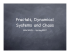More information

### Fractal Dimensions and the Sierpinski Tetrahedron (Proposal Draft)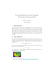More information

### Document 6520178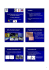More information

### Fractal Analysis of geomorphologic processes in the Linux environment using SCILAB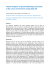More information

### (Microsoft PowerPoint - La complessit\340 della realt\340 biologica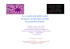More information

### PP-9. Follow the Energy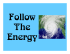More information

### Quantitative Description of Genomic Evolution of Olfactory Receptors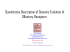More information

### Learning-based authentication of Jackson Pollock`s paintings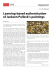More information

### Fractal Terrain Generation and Erosion for Playful Applications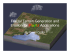More information

### Creating Fractals - MyopicBadger Fractals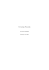More information

### Fractal Dimension in Butterflies` Wings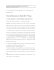More information

### Exploring the Effect of Direction on Vector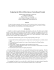More information

### Instructions for completing the Labs using F4e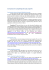More information

### Fractals and 3D printing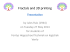More information

### Kaolin fractal dimension. Comparison with other properties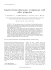More information

### Perceptual and Physiological Responses to the Visual Complexity of R.P. Taylor,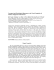More information

### Chapter 8 Fractal properties of plants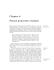More information

### 274 THE FRACTAL DIMENSION OF QUERCUS PETRAEA LEAVES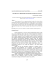More information

### World of Fractal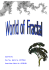More information

### Size and Shape, Scale and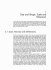More information

### A short history of fractal-Cantorian space-time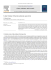More information

### Fractal Pack- educator`s guide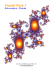More information